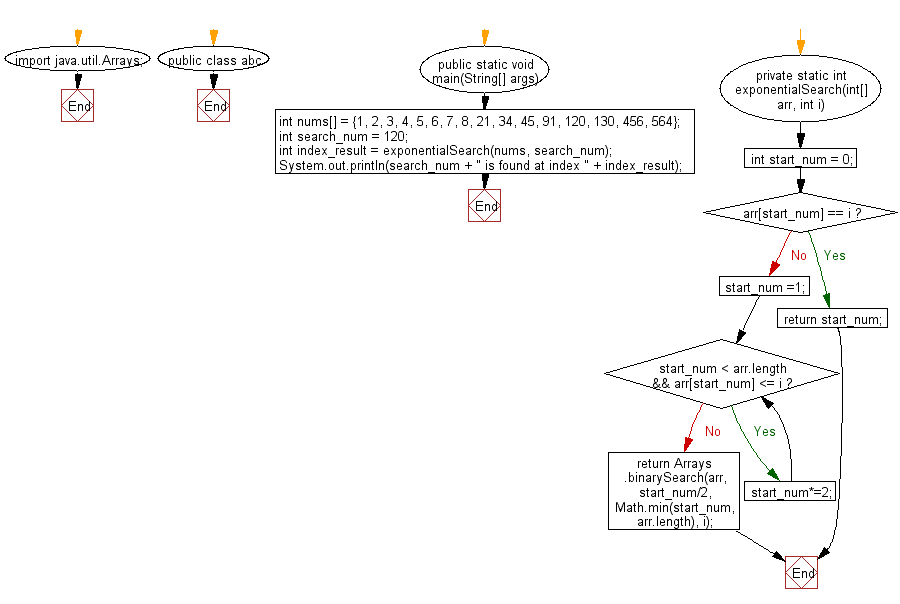﻿ Java exercises: Find a specified element in a given sorted array of elements using Exponential search - w3resource# Java Exercises: Find a specified element in a given sorted array of elements using Exponential search

## Java Search: Exercise-5 with Solution

Write a Java program to find a specified element in a given sorted array of elements using Exponential search.

From Wikipedia, in computer science, an exponential search (also called doubling search or galloping search or Struzik search) is an algorithm, created by Jon Bentley and Andrew Chi-Chih Yao in 1976, for searching sorted, unbounded/infinite lists. There are numerous ways to implement this with the most common being to determine a range that the search key resides in and performing a binary search within that range. This takes O(log i) where i is the position of the search key in the list, if the search key is in the list, or the position where the search key should be, if the search key is not in the list.

Sample Solution:

Java Code:

``````import java.util.Arrays;
public class abc {
public static void main(String[] args) {
int nums[] = {1, 2, 3, 4, 5, 6, 7, 8, 21, 34, 45, 91, 120, 130, 456, 564};
int search_num = 120;

// Find the index of searched item
int index_result = exponentialSearch(nums, search_num);

System.out.println(search_num + " is found at index " + index_result);

}

private static int exponentialSearch(int[] arr, int i) {
int start_num = 0;

if(arr[start_num] == i)
return start_num;
start_num =1;
while(start_num < arr.length && arr[start_num] <= i) {
start_num*=2;
}
return Arrays.binarySearch(arr, start_num/2, Math.min(start_num, arr.length), i);
}
}
```
```

Sample Output:

```120 is found at index 12
```

Flowchart:Java Code Editor:

What is the difficulty level of this exercise?

﻿

## Java: Tips of the Day

Array vs ArrayLists:

The main difference between these two is that an Array is of fixed size so once you have created an Array you cannot change it but the ArrayList is not of fixed size. You can create instances of ArrayLists without specifying its size. So if you create such instances of an ArrayList without specifying its size Java will create an instance of an ArrayList of default size.

Once an ArrayList is full it re-sizes itself. In fact, an ArrayList is internally supported by an array. So when an ArrayList is resized it will slow down its performance a bit as the contents of the old Array must be copied to a new Array.

At the same time, it's compulsory to specify the size of an Array directly or indirectly while creating it. And also Arrays can store both primitives and objects while ArrayLists only can store objects.

Ref: https://bit.ly/3o8L2KH